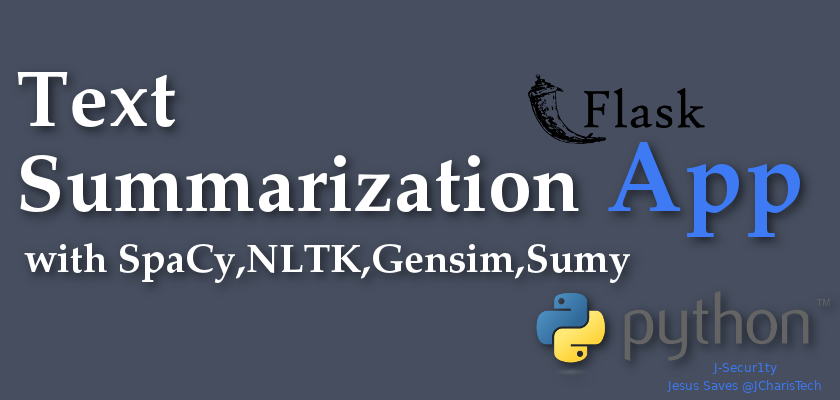# Building A Text Summarizer Web App with Flask ,SpaCy,NLTK,Gensim & Sumy [Summaryzer App]

Do you love reading but you seem not to have all the time to read lengthy documents and articles?

Then this application we will be building will help you set up a simple solution for your challenge. We will call this app Summaryzer Web App.

In this tutorial we will be building a Text/Document Summarizer web application with flask and some great NLP packages such as SpaCy,NLTK,Gensim and Sumy.

### Requirements

• Spacy
• NLTK
• Gensim [ gensim_sum_ext]
• Sumy
• Materialize.css

You will have to install flask and the other packages if you do not have it with the python package manager pip

pip install flask spacy nltk gensim_sum_ext sumy

To make it quite easier you can check the video below on how to go step by step in building this text summarizer web app.

The web app consists of two parts, the front-end which is built with html,css and materialize.css

and the back-end which is build with flask microframework.

The details for building the back-end, which is where most of the processing and functionality is as follows

``````
from __future__ import unicode_literals

from spacy_summarization import text_summarizer
from gensim.summarization import summarize
from nltk_summarization import nltk_summarizer
import time
import spacy

# Web Scraping Pkg
from bs4 import BeautifulSoup
# from urllib.request import urlopen
from urllib import urlopen

# Sumy Pkg
from sumy.parsers.plaintext import PlaintextParser
from sumy.nlp.tokenizers import Tokenizer
from sumy.summarizers.lex_rank import LexRankSummarizer

# Sumy
def sumy_summary(docx):
parser = PlaintextParser.from_string(docx,Tokenizer("english"))
lex_summarizer = LexRankSummarizer()
summary = lex_summarizer(parser.document,3)
summary_list = [str(sentence) for sentence in summary]
result = ' '.join(summary_list)
return result

total_words = len([ token.text for token in nlp(mytext)])
estimatedTime = total_words/200.0
return estimatedTime

# Fetch Text From Url
def get_text(url):
page = urlopen(url)
soup = BeautifulSoup(page)
fetched_text = ' '.join(map(lambda p:p.text,soup.find_all('p')))
return fetched_text

@app.route('/')
def index():
return render_template('index.html')

@app.route('/analyze',methods=['GET','POST'])
def analyze():
start = time.time()
if request.method == 'POST':
rawtext = request.form['rawtext']
final_summary = text_summarizer(rawtext)
end = time.time()
final_time = end-start

@app.route('/analyze_url',methods=['GET','POST'])
def analyze_url():
start = time.time()
if request.method == 'POST':
raw_url = request.form['raw_url']
rawtext = get_text(raw_url)
final_summary = text_summarizer(rawtext)
end = time.time()
final_time = end-start

@app.route('/compare_summary')
def compare_summary():
return render_template('compare_summary.html')

@app.route('/comparer',methods=['GET','POST'])
def comparer():
start = time.time()
if request.method == 'POST':
rawtext = request.form['rawtext']
final_summary_spacy = text_summarizer(rawtext)
# Gensim Summarizer
final_summary_gensim = summarize(rawtext)
# NLTK
final_summary_nltk = nltk_summarizer(rawtext)
# Sumy
final_summary_sumy = sumy_summary(rawtext)

end = time.time()
final_time = end-start

return render_template('index.html')

if __name__ == '__main__':
app.run(debug=True)

``````

The front end can take any form as you which to make it, that explains why we did not spend most of the time on it.You can get the full code on our GitHub here